## 常用连续型分布介绍及R语言实现

R的极客理想系列文章，涵盖了R的思想，使用，工具，创新等的一系列要点，以我个人的学习和体验去诠释R的强大。

R语言作为统计学一门语言，一直在小众领域闪耀着光芒。直到大数据的爆发，R语言变成了一门炙手可热的数据分析的利器。随着越来越多的工程背景的人的加入，R语言的社区在迅速扩大成长。现在已不仅仅是统计领域，教育，银行，电商，互联网….都在使用R语言。

• 张丹(Conan), 程序员Java,R,PHP,Javascript
• weibo：@Conan_Z
• blog: http://blog.fens.me
• email: bsspirit@gmail.com

http://blog.fens.me/r-density/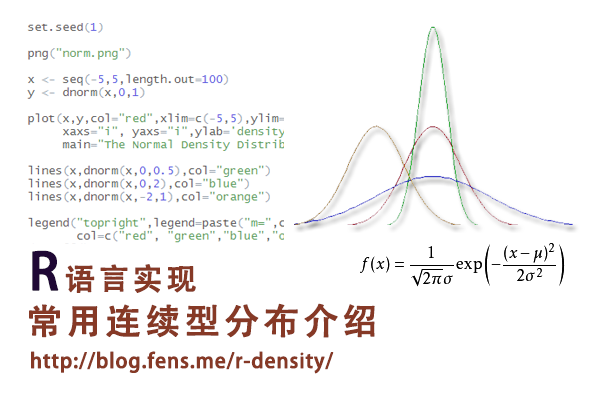1. 正态分布
2. 指数分步
3. γ(伽玛)分布
4. weibull分布
5. F分布
6. T分布
7. β(贝塔)分布
8. χ²(卡方)分布
9. 均匀分布

## 1. 正态分布

1). 概率密度函数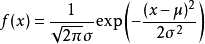set.seed(1)
x <- seq(-5,5,length.out=100)
y <- dnorm(x,0,1)

plot(x,y,col="red",xlim=c(-5,5),ylim=c(0,1),type='l',
xaxs="i", yaxs="i",ylab='density',xlab='',
main="The Normal Density Distribution")

lines(x,dnorm(x,0,0.5),col="green")
lines(x,dnorm(x,0,2),col="blue")
lines(x,dnorm(x,-2,1),col="orange")

legend("topright",legend=paste("m=",c(0,0,0,-2)," sd=", c(1,0.5,2,1)), lwd=1, col=c("red", "green","blue","orange"))2). 累积分布函数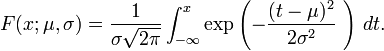set.seed(1)
x <- seq(-5,5,length.out=100)
y <- pnorm(x,0,1)

plot(x,y,col="red",xlim=c(-5,5),ylim=c(0,1),type='l',
xaxs="i", yaxs="i",ylab='density',xlab='',
main="The Normal Cumulative Distribution")

lines(x,pnorm(x,0,0.5),col="green")
lines(x,pnorm(x,0,2),col="blue")
lines(x,pnorm(x,-2,1),col="orange")

legend("bottomright",legend=paste("m=",c(0,0,0,-2)," sd=", c(1,0.5,2,1)), lwd=1,col=c("red", "green","blue","orange"))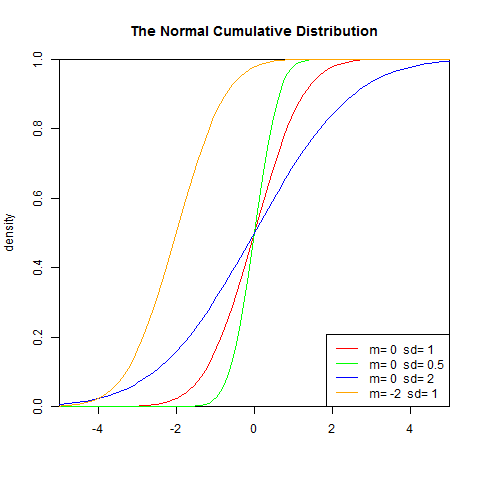3). 分布检验

Shapiro-Wilk正态分布检验: 用来检验是否数据符合正态分布，类似于线性回归的方法一样，是检验其于回归曲线的残差。该方法推荐在样本量很小的时候使用，样本在3到5000之间。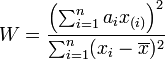• 统计量W 最大值是1，越接近1，表示样本与正态分布匹配
• p值，如果p-value小于显著性水平α(0.05)，则拒绝H0
• R语言程序


> set.seed(1)
> S<-rnorm(1000)
> shapiro.test(S)
Shapiro-Wilk normality test
data:  S
W = 0.9988, p-value = 0.7256


结论: W接近1，p-value>0.05，不能拒绝原假设，所以数据集S符合正态分布！

Kolmogorov-Smirnov连续分布检验:检验单一样本是不是服从某一预先假设的特定分布的方法。以样本数据的累计频数分布与特定理论分布比较，若两者间的差距很小，则推论该样本取自某特定分布族。

该检验原假设为H0:数据集符合正态分布，H1:样本所来自的总体分布不符合正态分布。令F0(x)表示预先假设的理论分布，Fn(x)表示随机样本的累计概率(频率)函数.

统计量D为: D=max|F0(x) - Fn(x)|

• D值越小，越接近0，表示样本数据越接近正态分布
• p值，如果p-value小于显著性水平α(0.05)，则拒绝H0

> set.seed(1)
> S<-rnorm(1000)
> ks.test(S, "pnorm")
One-sample Kolmogorov-Smirnov test
data:  S
D = 0.0211, p-value = 0.7673
alternative hypothesis: two-sided


结论: D值很小, p-value>0.05，不能拒绝原假设，所以数据集S符合正态分布！

## 2. 指数分布

指数分布(Exponential distribution)用来表示独立随机事件发生的时间间隔，比如旅客进机场的时间间隔、中文维基百科新条目出现的时间间隔等等。

许多电子产品的寿命分布一般服从指数分布。有的系统的寿命分布也可用指数分布来近似。它在可靠性研究中是最常用的一种分布形式。指数分布是伽玛分布和weibull分布的特殊情况，产品的失效是偶然失效时，其寿命服从指数分布。

指数分布可以看作当weibull分布中的形状系数等于1的特殊分布，指数分布的失效率是与时间t无关的常数，所以分布函数简单。

1). 概率密度函数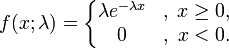其中λ > 0是分布的一个参数，常被称为率参数（rate parameter）。即每单位时间发生该事件的次数。指数分布的区间是[0,∞)。 如果一个随机变量X 呈指数分布，则可以写作：X ~ Exponential（λ）。


set.seed(1)
x<-seq(-1,2,length.out=100)
y<-dexp(x,0.5)

plot(x,y,col="red",xlim=c(0,2),ylim=c(0,5),type='l',
xaxs="i", yaxs="i",ylab='density',xlab='',
main="The Exponential Density Distribution")
lines(x,dexp(x,1),col="green")
lines(x,dexp(x,2),col="blue")
lines(x,dexp(x,5),col="orange")

legend("topright",legend=paste("rate=",c(.5, 1, 2,5)), lwd=1,col=c("red", "green","blue","orange"))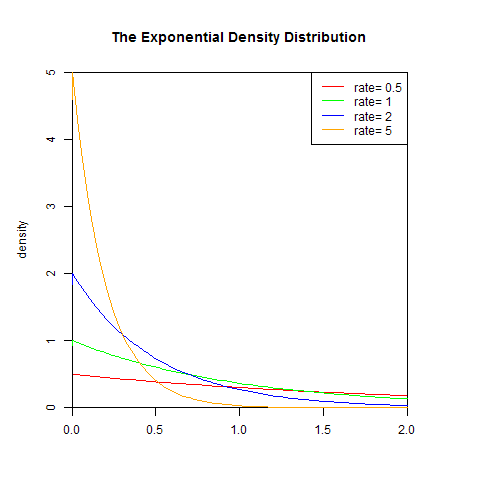2). 累积分布函数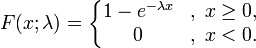set.seed(1)
x<-seq(-1,2,length.out=100)
y<-pexp(x,0.5)

plot(x,y,col="red",xlim=c(0,2),ylim=c(0,1),type='l',
xaxs="i", yaxs="i",ylab='density',xlab='',
main="The Exponential Cumulative Distribution Function")
lines(x,pexp(x,1),col="green")
lines(x,pexp(x,2),col="blue")
lines(x,pexp(x,5),col="orange")

legend("bottomright",legend=paste("rate=",c(.5, 1, 2,5)), lwd=1, col=c("red", "green","blue","orange"))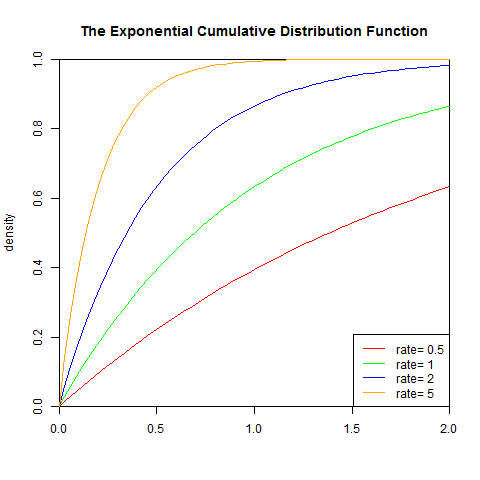3). 分布检验

Kolmogorov-Smirnov连续分布检验:检验单一样本是不是服从某一预先假设的特定分布的方法。以样本数据的累计频数分布与特定理论分布比较，若两者间的差距很小，则推论该样本取自某特定分布族。

该检验原假设为H0:数据集符合指数分布，H1:样本所来自的总体分布不符合指数分布。令F0(x)表示预先假设的理论分布，Fn(x)表示随机样本的累计概率(频率)函数.

统计量D为: D=max|F0(x) - Fn(x)|

• D值越小，越接近0，表示样本数据越接近指数分布
• p值，如果p-value小于显著性水平α(0.05)，则拒绝H0

> set.seed(1)
> S<-rexp(1000)
> ks.test(S, "pexp")
One-sample Kolmogorov-Smirnov test
data:  S
D = 0.0387, p-value = 0.1001
alternative hypothesis: two-sided


结论: D值很小, p-value>0.05，不能拒绝原假设，所以数据集S符合指数分布！

## 3. γ(伽玛)分布

伽玛分布(Gamma)是著名的皮尔逊概率分布函数簇中的重要一员，称为皮尔逊Ⅲ型分布。它的曲线有一个峰，但左右不对称。

伽玛分布中的参数α，称为形状参数，β称为尺度参数。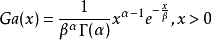伽玛函数为：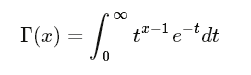伽玛函数是阶乘在实数上的泛化。

1). 概率密度函数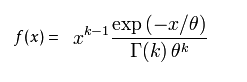set.seed(1)
x<-seq(0,10,length.out=100)
y<-dgamma(x,1,2)

plot(x,y,col="red",xlim=c(0,10),ylim=c(0,2),type='l',
xaxs="i", yaxs="i",ylab='density',xlab='',
main="The Gamma Density Distribution")

lines(x,dgamma(x,2,2),col="green")
lines(x,dgamma(x,3,2),col="blue")
lines(x,dgamma(x,5,1),col="orange")
lines(x,dgamma(x,9,1),col="black")

legend("topright",legend=paste("shape=",c(1,2,3,5,9)," rate=", c(2,2,2,1,1)), lwd=1, col=c("red", "green","blue","orange","black"))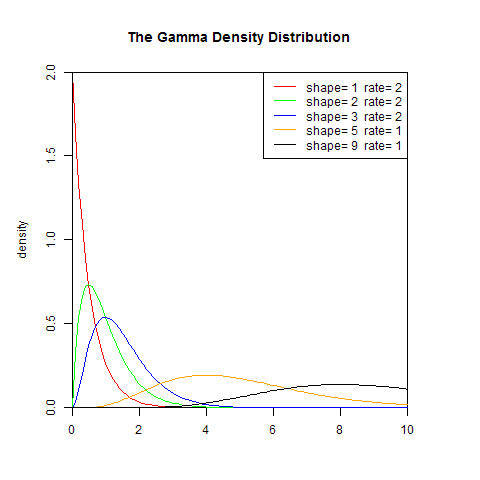2). 累积分布函数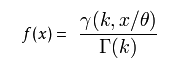set.seed(1)
x<-seq(0,10,length.out=100)
y<-pgamma(x,1,2)

plot(x,y,col="red",xlim=c(0,10),ylim=c(0,1),type='l',
xaxs="i", yaxs="i",ylab='density',xlab='',
main="The Gamma Cumulative Distribution Function")

lines(x,pgamma(x,2,2),col="green")
lines(x,pgamma(x,3,2),col="blue")
lines(x,pgamma(x,5,1),col="orange")
lines(x,pgamma(x,9,1),col="black")

legend("bottomright",legend=paste("shape=",c(1,2,3,5,9)," rate=", c(2,2,2,1,1)), lwd=1, col=c("red", "green","blue","orange","black"))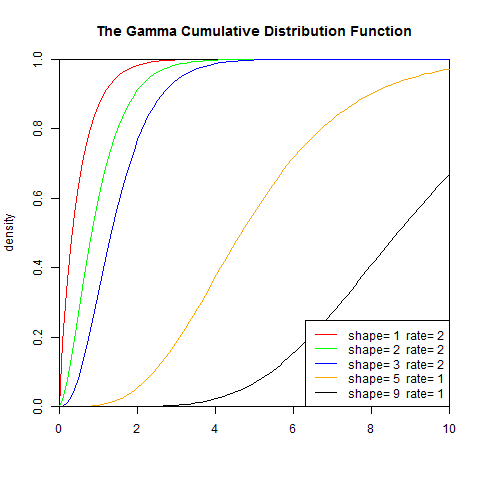3). 分布检验

Kolmogorov-Smirnov连续分布检验: 检验单一样本是不是服从某一预先假设的特定分布的方法。以样本数据的累计频数分布与特定理论分布比较，若两者间的差距很小，则推论该样本取自某特定分布族。

该检验原假设为H0:数据集符合伽玛分布，H1:样本所来自的总体分布不符合伽玛分布。令F0(x)表示预先假设的理论分布，Fn(x)表示随机样本的累计概率(频率)函数.

统计量D为: D=max|F0(x) - Fn(x)|

• D值越小，越接近0，表示样本数据越接近伽玛分布
• p值，如果p-value小于显著性水平α(0.05)，则拒绝H0

> set.seed(1)
> S<-rgamma(1000,1)
> ks.test(S, "pgamma", 1)
One-sample Kolmogorov-Smirnov test
data:  S
D = 0.0363, p-value = 0.1438
alternative hypothesis: two-sided


结论: D值很小, p-value>0.05，不能拒绝原假设，所以数据集S符合shape=1伽玛分布！

检验失败：


> ks.test(S, "pgamma", 2)
One-sample Kolmogorov-Smirnov test
data:  S
D = 0.3801, p-value < 2.2e-16
alternative hypothesis: two-sided


结论:D值不够小, p-value<0.05，拒绝原假设，所以数据集S符合shape=2伽玛分布！

## 4. weibull分布

weibull(韦伯)分布，又称韦氏分布或威布尔分布，是可靠性分析和寿命检验的理论基础。Weibull分布能被应用于很多形式，分布由形状、尺度（范围）和位置三个参数决定。其中形状参数是最重要的参数，决定分布密度曲线的基本形状，尺度参数起放大或缩小曲线的作用，但不影响分布的形状。

Weibull分布通常用在故障分析领域( field of failure analysis)中；尤其是它可以模拟(mimic) 故障率(failture rate)持续( over time)变化的分布。故障率为：

• 一直为常量(constant over time)， 那么 α = 1， 暗示在随机事件中发生
• 一直减少(decreases over time)，那么α < 1， 暗示"早期失效(infant mortality)"
• 一直增加(increases over time)，那么α > 1， 暗示"耗尽(wear out)" - 随着时间的推进，失败的可能性变大

1). 概率密度函数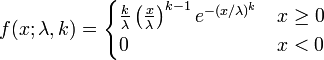set.seed(1)
x<- seq(0, 2.5, length.out=1000)
y<- dweibull(x, 0.5)

plot(x, y, type="l", col="blue",xlim=c(0, 2.5),ylim=c(0, 6),
xaxs="i", yaxs="i",ylab='density',xlab='',
main="The Weibull Density Distribution")

lines(x, dweibull(x, 1), type="l", col="red")
lines(x, dweibull(x, 1.5), type="l", col="magenta")
lines(x, dweibull(x, 5), type="l", col="green")
lines(x, dweibull(x, 15), type="l", col="purple")
legend("topright", legend=paste("shape =", c(.5, 1, 1.5, 5, 15)), lwd=1,col=c("blue", "red", "magenta", "green","purple"))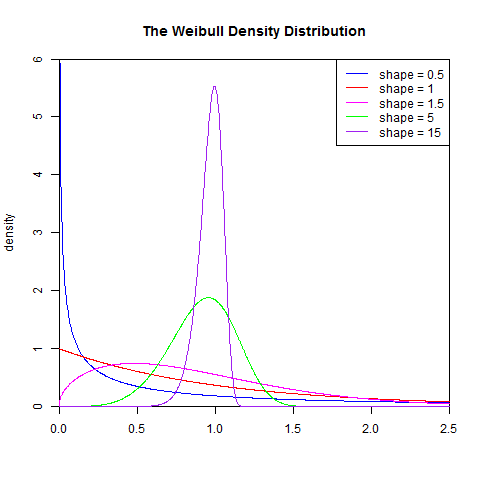2). 累积分布函数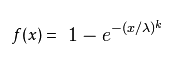set.seed(1)
x<- seq(0, 2.5, length.out=1000)
y<- pweibull(x, 0.5)

plot(x, y, type="l", col="blue",xlim=c(0, 2.5),ylim=c(0, 1.2),
xaxs="i", yaxs="i",ylab='density',xlab='',
main="The Weibull Cumulative Distribution Function")

lines(x, pweibull(x, 1), type="l", col="red")
lines(x, pweibull(x, 1.5), type="l", col="magenta")
lines(x, pweibull(x, 5), type="l", col="green")
lines(x, pweibull(x, 15), type="l", col="purple")
legend("bottomright", legend=paste("shape =", c(.5, 1, 1.5, 5, 15)), lwd=1, col=c("blue", "red", "magenta", "green","purple"))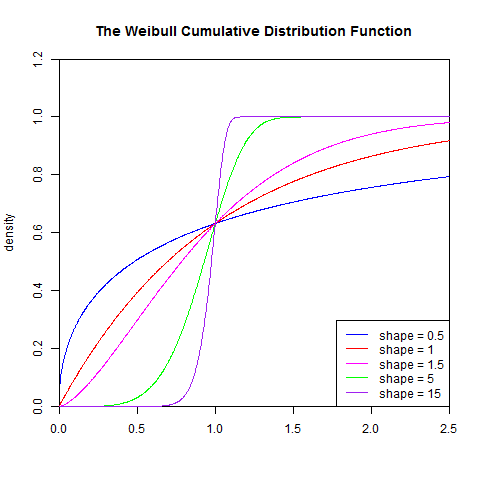3). 分布检验

Kolmogorov-Smirnov连续分布检验: 检验单一样本是不是服从某一预先假设的特定分布的方法。以样本数据的累计频数分布与特定理论分布比较，若两者间的差距很小，则推论该样本取自某特定分布族。

该检验原假设为H0:数据集符合weibull分布，H1:样本所来自的总体分布不符合weibull分布。令F0(x)表示预先假设的理论分布，Fn(x)表示随机样本的累计概率(频率)函数.

统计量D为: D=max|F0(x) - Fn(x)|

• D值越小，越接近0，表示样本数据越接近weibull分布
• p值，如果p-value小于显著性水平α(0.05)，则拒绝H0

> set.seed(1)
> S<-rweibull(1000,1)
> ks.test(S, "pweibull",1)
One-sample Kolmogorov-Smirnov test
data:  S
D = 0.0244, p-value = 0.5928
alternative hypothesis: two-sided


结论: D值很小, p-value>0.05，不能拒绝原假设，所以数据集S符合shape=1的weibull分布！

## 5. F分布

F-分布（F-distribution）是一种连续概率分布，被广泛应用于似然比率检验，特别是ANOVA中。F分布定义为：设X、Y为两个独立的随机变量，X服从自由度为k1的卡方分布，Y服从自由度为k2的卡方分布，这2 个独立的卡方分布被各自的自由度除以后的比率这一统计量的分布。即： 上式F服从第一自由度为k1，第二自由度为k2的F分布。

F分布的性质

• 它是一种非对称分布
• 它有两个自由度，即n1 -1和n2-1，相应的分布记为F（ n1 –1， n2-1）， n1 –1通常称为分子自由度， n2-1通常称为分母自由度
• F分布是一个以自由度n1 –1和n2-1为参数的分布族，不同的自由度决定了F 分布的形状
• F分布的倒数性质：Fα,df1,df2=1/F1-α,df1,df2

1). 概率密度函数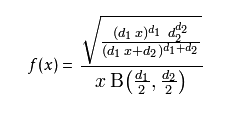B是Beta函数(beta function)


set.seed(1)
x<-seq(0,5,length.out=1000)
y<-df(x,1,1,0)

plot(x,y,col="red",xlim=c(0,5),ylim=c(0,1),type='l',
xaxs="i", yaxs="i",ylab='density',xlab='',
main="The F Density Distribution")

lines(x,df(x,1,1,2),col="green")
lines(x,df(x,2,2,2),col="blue")
lines(x,df(x,2,4,4),col="orange")

legend("topright",legend=paste("df1=",c(1,1,2,2),"df2=",c(1,1,2,4)," ncp=", c(0,2,2,4)), lwd=1, col=c("red", "green","blue","orange"))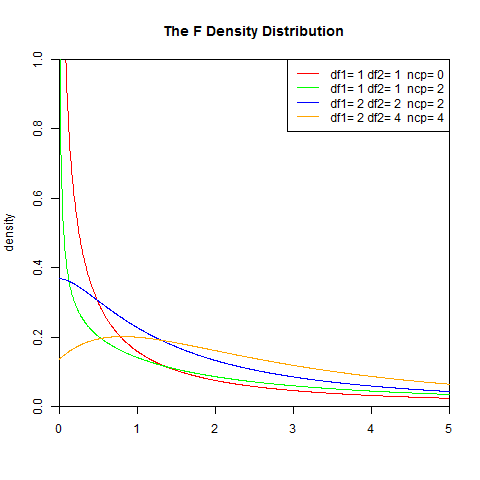2). 累积分布函数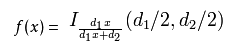I是不完全Beta函数


set.seed(1)
x<-seq(0,5,length.out=1000)
y<-df(x,1,1,0)

plot(x,y,col="red",xlim=c(0,5),ylim=c(0,1),type='l',
xaxs="i", yaxs="i",ylab='density',xlab='',
main="The F Cumulative Distribution Function")

lines(x,pf(x,1,1,2),col="green")
lines(x,pf(x,2,2,2),col="blue")
lines(x,pf(x,2,4,4),col="orange")

legend("topright",legend=paste("df1=",c(1,1,2,2),"df2=",c(1,1,2,4)," ncp=", c(0,2,2,4)), lwd=1, col=c("red", "green","blue","orange"))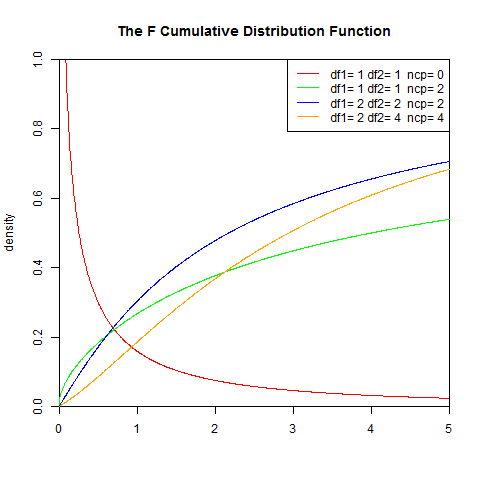3). 分布检验

Kolmogorov-Smirnov连续分布检验: 检验单一样本是不是服从某一预先假设的特定分布的方法。以样本数据的累计频数分布与特定理论分布比较，若两者间的差距很小，则推论该样本取自某特定分布族。

该检验原假设为H0:数据集符合F分布，H1:样本所来自的总体分布不符合F分布。令F0(x)表示预先假设的理论分布，Fn(x)表示随机样本的累计概率(频率)函数.

统计量D为: D=max|F0(x) - Fn(x)|

• D值越小，越接近0，表示样本数据越接近F分布
• p值，如果p-value小于显著性水平α(0.05)，则拒绝H0

> set.seed(1)
> S<-rf(1000,1,1,2)
> ks.test(S, "pf", 1,1,2)
One-sample Kolmogorov-Smirnov test
data:  S
D = 0.0113, p-value = 0.9996
alternative hypothesis: two-sided


结论: D值很小, p-value>0.05，不能拒绝原假设，所以数据集S符合df1=1, df2=1, ncp=2的F分布！

## 6. T分布

学生t-分布（Student's t-distribution），可简称为t分布。应用在估计呈正态分布的母群体之平均数。它是对两个样本均值差异进行显著性测试的学生t检定的基础。学生t检定改进了Z检定（Z-test），因为Z检定以母体标准差已知为前提。虽然在样本数量大（超过30个）时，可以应用Z检定来求得近似值，但Z检定用在小样本会产生很大的误差，因此必须改用学生t检定以求准确。

在母体标准差未知的情况下，不论样本数量大或小皆可应用学生t检定。在待比较的数据有三组以上时，因为误差无法压低，此时可以用变异数分析（ANOVA）代替学生t检定。

1). 概率密度函数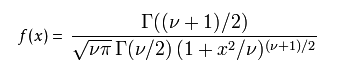v 等于n − 1。 T的分布称为t-分布。参数\nu 一般被称为自由度。
γ 是伽玛函数。


set.seed(1)
x<-seq(-5,5,length.out=1000)
y<-dt(x,1,0)

plot(x,y,col="red",xlim=c(-5,5),ylim=c(0,0.5),type='l',
xaxs="i", yaxs="i",ylab='density',xlab='',
main="The T Density Distribution")

lines(x,dt(x,5,0),col="green")
lines(x,dt(x,5,2),col="blue")
lines(x,dt(x,50,4),col="orange")

legend("topleft",legend=paste("df=",c(1,5,5,50)," ncp=", c(0,0,2,4)), lwd=1, col=c("red", "green","blue","orange"))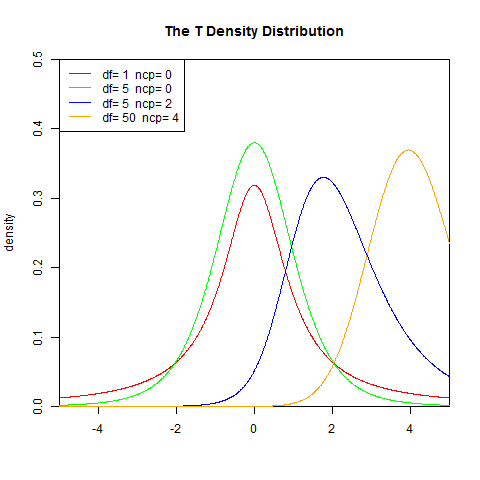2). 累积分布函数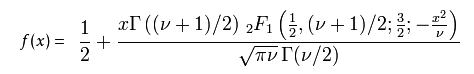v 等于n − 1。 T的分布称为t-分布。参数\nu 一般被称为自由度。
γ 是伽玛函数。


set.seed(1)
x<-seq(-5,5,length.out=1000)
y<-pt(x,1,0)

plot(x,y,col="red",xlim=c(-5,5),ylim=c(0,0.5),type='l',
xaxs="i", yaxs="i",ylab='density',xlab='',
main="The T Cumulative Distribution Function")

lines(x,pt(x,5,0),col="green")
lines(x,pt(x,5,2),col="blue")
lines(x,pt(x,50,4),col="orange")

legend("topleft",legend=paste("df=",c(1,5,5,50)," ncp=", c(0,0,2,4)), lwd=1, col=c("red", "green","blue","orange"))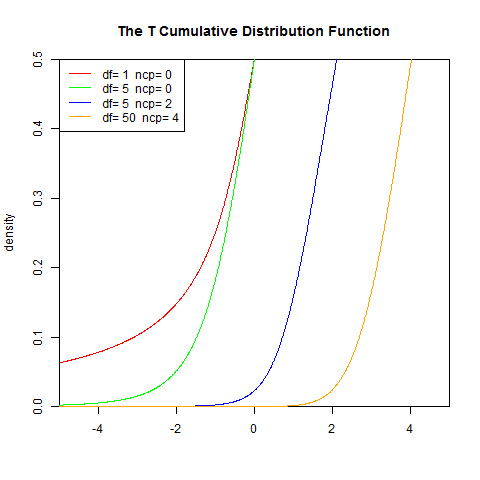3). 分布检验

Kolmogorov-Smirnov连续分布检验: 检验单一样本是不是服从某一预先假设的特定分布的方法。以样本数据的累计频数分布与特定理论分布比较，若两者间的差距很小，则推论该样本取自某特定分布族。

该检验原假设为H0:数据集符合T分布，H1:样本所来自的总体分布不符合T分布。令F0(x)表示预先假设的理论分布，Fn(x)表示随机样本的累计概率(频率)函数.

统计量D为: D=max|F0(x) - Fn(x)|

• D值越小，越接近0，表示样本数据越接近T分布
• p值，如果p-value小于显著性水平α(0.05)，则拒绝H0

> set.seed(1)
> S<-rt(1000, 1,2)
> ks.test(S, "pt", 1, 2)
One-sample Kolmogorov-Smirnov test
data:  S
D = 0.0253, p-value = 0.5461
alternative hypothesis: two-sided


结论: D值很小, p-value>0.05，不能拒绝原假设，所以数据集S符合df1=1, ncp=2的T分布！

## 7. β(贝塔Beta)分布

贝塔分布(Beta Distribution)是指一组定义在(0,1)区间的连续概率分布，Beta分布有α和β两个参数α,β>0，其中α为成功次数加1，β为失败次数加1。

Beta分布的一个重要应该是作为伯努利分布和二项式分布的共轭先验分布出现，在机器学习和数理统计学中有重要应用。贝塔分布中的参数可以理解为伪计数，伯努利分布的似然函数可以表示为，表示一次事件发生的概率，它为贝塔有相同的形式，因此可以用贝塔分布作为其先验分布。

1). 概率密度函数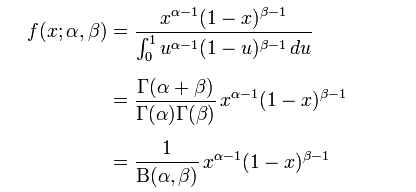随机变量X服从参数为a, β，服从Beta分布
γ 是伽玛函数


set.seed(1)
x<-seq(-5,5,length.out=10000)
y<-dbeta(x,0.5,0.5)

plot(x,y,col="red",xlim=c(0,1),ylim=c(0,6),type='l',
xaxs="i", yaxs="i",ylab='density',xlab='',
main="The Beta Density Distribution")

lines(x,dbeta(x,5,1),col="green")
lines(x,dbeta(x,1,3),col="blue")
lines(x,dbeta(x,2,2),col="orange")
lines(x,dbeta(x,2,5),col="black")

legend("top",legend=paste("a=",c(.5,5,1,2,2)," b=", c(.5,1,3,2,5)), lwd=1,col=c("red", "green","blue","orange","black"))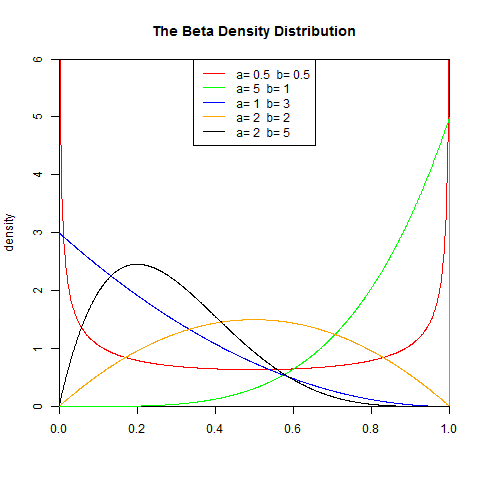2). 累积分布函数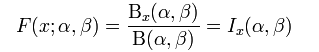I是正则不完全Beta函数


set.seed(1)
x<-seq(-5,5,length.out=10000)
y<-pbeta(x,0.5,0.5)

plot(x,y,col="red",xlim=c(0,1),ylim=c(0,1),type='l',
xaxs="i", yaxs="i",ylab='density',xlab='',
main="The Beta Cumulative Distribution Function")

lines(x,pbeta(x,5,1),col="green")
lines(x,pbeta(x,1,3),col="blue")
lines(x,pbeta(x,2,2),col="orange")
lines(x,pbeta(x,2,5),col="black")

legend("topleft",legend=paste("a=",c(.5,5,1,2,2)," b=", c(.5,1,3,2,5)), lwd=1,col=c("red", "green","blue","orange","black"))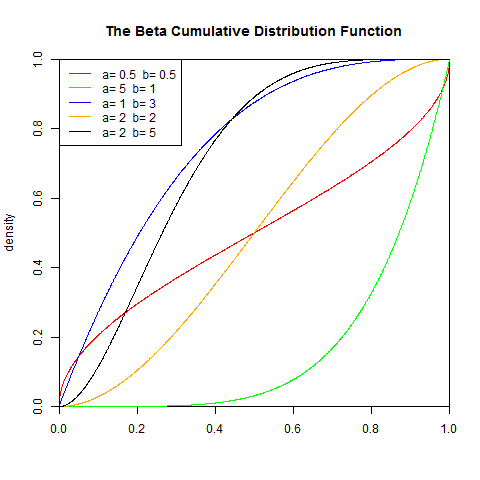3). 分布检验

Kolmogorov-Smirnov连续分布检验: 检验单一样本是不是服从某一预先假设的特定分布的方法。以样本数据的累计频数分布与特定理论分布比较，若两者间的差距很小，则推论该样本取自某特定分布族。

该检验原假设为H0:数据集符合Beta分布，H1:样本所来自的总体分布不符合Beta分布。令F0(x)表示预先假设的理论分布，Fn(x)表示随机样本的累计概率(频率)函数.

统计量D为: D=max|F0(x) - Fn(x)|

• D值越小，越接近0，表示样本数据越接近Beta分布
• p值，如果p-value小于显著性水平α(0.05)，则拒绝H0

> set.seed(1)
> S<-rbeta(1000,1,2)
> ks.test(S, "pbeta",1,2)
One-sample Kolmogorov-Smirnov test
data:  S
D = 0.0202, p-value = 0.807
alternative hypothesis: two-sided


结论: D值很小, p-value>0.05，不能拒绝原假设，所以数据集S符合shape1=1, shape2=2的Beta分布！

## 8. χ²(卡方)分布

若n个相互独立的随机变量ξ₁、ξ₂、……、ξn ，均服从标准正态分布（也称独立同分布于标准正态分布），则这n个服从标准正态分布的随机变量的平方和构成一新的随机变量，其分布规律称为χ²分布（chi-square distribution）。其中参数n称为自由度，自由度不同就是另一个χ²分布，正如正态分布中均值或方差不同就是另一个正态分布一样。

1). 概率密度函数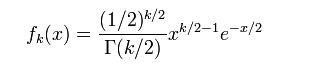γ 是伽玛函数


set.seed(1)
x<-seq(0,10,length.out=1000)
y<-dchisq(x,1)

plot(x,y,col="red",xlim=c(0,5),ylim=c(0,2),type='l',
xaxs="i", yaxs="i",ylab='density',xlab='',
main="The Chisq Density Distribution")

lines(x,dchisq(x,2),col="green")
lines(x,dchisq(x,3),col="blue")
lines(x,dchisq(x,10),col="orange")

legend("topright",legend=paste("df=",c(1,2,3,10)), lwd=1, col=c("red", "green","blue","orange"))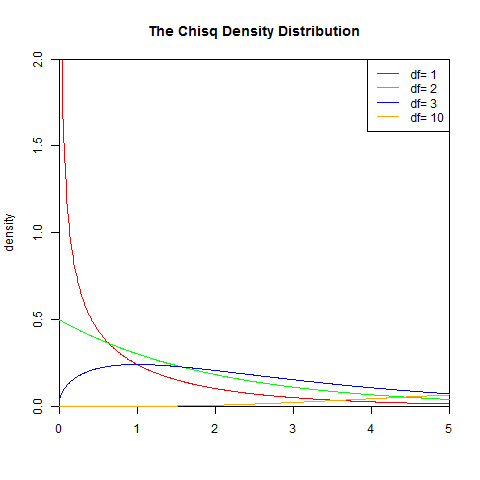2). 累积分布函数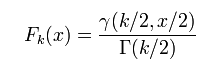γ 是伽玛函数


set.seed(1)
x<-seq(0,10,length.out=1000)
y<-pchisq(x,1)

plot(x,y,col="red",xlim=c(0,10),ylim=c(0,1),type='l',
xaxs="i", yaxs="i",ylab='density',xlab='',
main="The Chisq Cumulative Distribution Function")

lines(x,pchisq(x,2),col="green")
lines(x,pchisq(x,3),col="blue")
lines(x,pchisq(x,10),col="orange")

legend("topleft",legend=paste("df=",c(1,2,3,10)), lwd=1, col=c("red", "green","blue","orange"))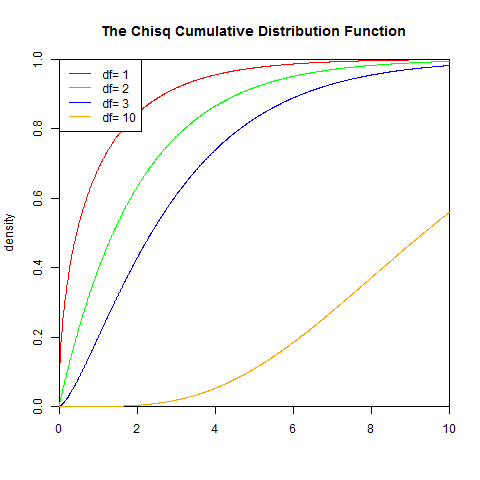3). 分布检验

Kolmogorov-Smirnov连续分布检验: 检验单一样本是不是服从某一预先假设的特定分布的方法。以样本数据的累计频数分布与特定理论分布比较，若两者间的差距很小，则推论该样本取自某特定分布族。

该检验原假设为H0:数据集符合卡方分布，H1:样本所来自的总体分布不符合卡方分布。令F0(x)表示预先假设的理论分布，Fn(x)表示随机样本的累计概率(频率)函数.

统计量D为: D=max|F0(x) - Fn(x)|

• D值越小，越接近0，表示样本数据越接近卡方分布
• p值，如果p-value小于显著性水平α(0.05)，则拒绝H0

> set.seed(1)
> S<-rchisq(1000,1)
> ks.test(S, "pchisq",1)
One-sample Kolmogorov-Smirnov test
data:  S
D = 0.0254, p-value = 0.5385
alternative hypothesis: two-sided


结论: D值很小, p-value>0.05，不能拒绝原假设，所以数据集S符合df=1的卡方分布！

## 9. 均匀分布

均匀分布(Uniform distribution)是均匀的，不偏差的一种简单的概率分布，分为：离散型均匀分布与连续型均匀分布。

1). 概率密度函数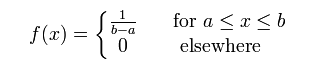set.seed(1)
x<-seq(0,10,length.out=1000)
y<-dunif(x,0,1)

plot(x,y,col="red",xlim=c(0,10),ylim=c(0,1.2),type='l',
xaxs="i", yaxs="i",ylab='density',xlab='',
main="The Uniform Density Distribution")
lines(x,dnorm(x,0,0.5),col="green")
lines(x,dnorm(x,0,2),col="blue")
lines(x,dnorm(x,-2,1),col="orange")
lines(x,dnorm(x,4,2),col="purple")

legend("topright",legend=paste("m=",c(0,0,0,-2,4)," sd=", c(1,0.5,2,1,2)), lwd=1, col=c("red", "green","blue","orange","purple"))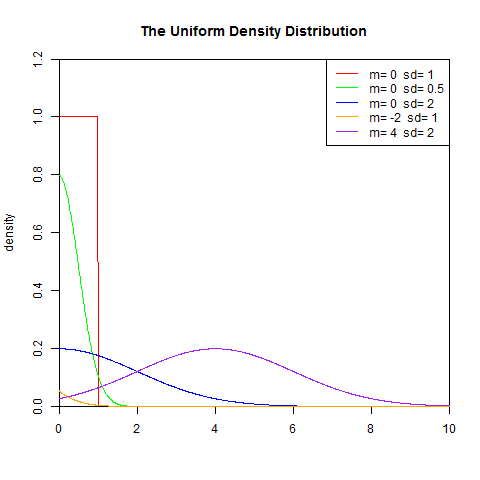2). 累积分布函数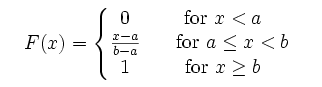set.seed(1)
x<-seq(0,10,length.out=1000)
y<-punif(x,0,1)

plot(x,y,col="red",xlim=c(0,10),ylim=c(0,1.2),type='l',
xaxs="i", yaxs="i",ylab='density',xlab='',
main="The Uniform Cumulative Distribution Function")

lines(x,punif(x,0,0.5),col="green")
lines(x,punif(x,0,2),col="blue")
lines(x,punif(x,-2,1),col="orange")

legend("bottomright",legend=paste("m=",c(0,0,0,-2)," sd=", c(1,0.5,2,1)), lwd=1, col=c("red", "green","blue","orange","purple"))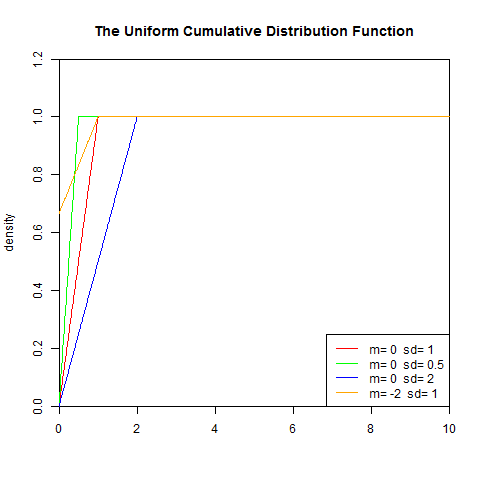3). 分布检验

Kolmogorov-Smirnov连续分布检验: 检验单一样本是不是服从某一预先假设的特定分布的方法。以样本数据的累计频数分布与特定理论分布比较，若两者间的差距很小，则推论该样本取自某特定分布族。

该检验原假设为H0:数据集符合均匀分布，H1:样本所来自的总体分布不符合均匀分布。令F0(x)表示预先假设的理论分布，Fn(x)表示随机样本的累计概率(频率)函数.

统计量D为: D=max|F0(x) - Fn(x)|

• D值越小，越接近0，表示样本数据越接近均匀分布
• p值，如果p-value小于显著性水平α(0.05)，则拒绝H0

> set.seed(1)
> S<-runif(1000)
> ks.test(S, "punif")
One-sample Kolmogorov-Smirnov test
data:  S
D = 0.0244, p-value = 0.5928
alternative hypothesis: two-sided


结论: D值很小, p-value>0.05，不能拒绝原假设，所以数据集S符合均匀分布！

在我们掌握了，这几种常用的连续型分布后，我们就可以基于这些分布来建模了，很多的算法模型就能解释通了！！

参考资料

转载请注明出处：
http://blog.fens.me/r-density/This entry was posted in R语言实践

Article Rating
Subscribe
Notify ofThis site uses Akismet to reduce spam. Learn how your comment data is processed.

Inline Feedbacks[…] 请参考文章：http://blog.fens.me/r-density/ […]http://finzi.psych.upenn.edu/library/rmutil/html/Levy.htmlhttp://finzi.psych.upenn.edu/library/rmutil/html/Levy.html[…] 对残差进行Shapiro-Wilk正态分布检验，W接近1，p-value>0.05，证明数据集符合正态分布！关于正态分布的介绍，请参考文章常用连续型分布介绍及R语言实现。 […][…] 统计分布，是用来判断数据是否是满足某种统计学分布，如果能够验证了，那么我们就可以用到这种分布的特性来理解我们的数据集的情况了。常见的连续型的统计分布有9种，其中最常用的就是正态分布的假设。关于统计分布的详细介绍，请参考文章 常用连续型分布介绍及R语言实现。 […]

14
0